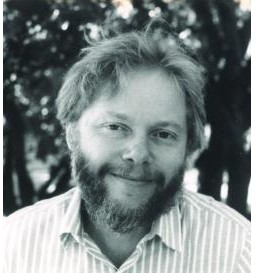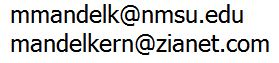Mark Mandelkern
prof. math. emer.
New Mexico State University

Constructive Geometry

Constructive harmonic conjugates, Beitr. Algebra Geom. 60 (2019), 391–398.   pdf   doi

A constructive version of the Sylvester-Gallai Theorem, Acta Math. Hungar. 150 (2016), 121-130.   pdf   doi

A constructive real projective plane, J. Geom. 107 (2016), 19-60.   pdf   doi
- Correction: see Constructive harmonic conjugates, above.

Constructive projective extension of an incidence plane, Trans. Amer. Math. Soc. 366 (2014), 691-706.   pdf

The common point problem in constructive projective geometry, Indag. Math. (N.S.) 24 (2013), 111–114.   pdf

Constructive coordinatization of Desarguesian planes, Beiträge Algebra Geom. 48 (2007), 547-589.   pdf

Constructive Mathematics

Constructive mathematics, Math. Mag. 58 (1985), 272-280. (expository)   pdf

Brouwerian counterexamples, Math. Mag. 62 (1989) 3-27. (expository/survey)   pdf

Constructive Analysis

Connectivity of an interval, Proc. Amer. Math. Soc. 54 (1976), 170-172.   pdf

An example in connectivity, Bull. London Math. Soc. 10 (1978), 314.   pdf

Suprema of located sets, J. London Math. Soc. (2) 20 (1979), 161-164.   pdf

Resolutions on the line, Pacific J. Math. 88 (1980), 91-100.   pdf

Located sets on the line, Pacific J. Math. 95 (1981), 401-409.   pdf

Continuity of monotone functions, Pacific J. Math. 99 (1982), 413-418.   pdf

Components of an open set, J. Austral. Math. Soc. (Series A) 33 (1982), 249-261.   pdf

Constructive Continuity, Memoirs Amer. Math. Soc. 42, nr.277, 1983.

Constructive irrational space, Manuscripta Math. 60 (1988), 397-406.   pdf   MR

Constructively complete finite sets, Z. Math. Logik Grund. Math. 34, (1988), 97-103.

Limited omniscience and the Bolzano-Weierstrass principle, Bull. London Math. Soc. 20 (1988), 319-320.   pdf

Metrization of the one-point compactification, Proc. Amer. Math. Soc. 107 (1989) 1111-1115.   pdf

Open subspaces of locally compact metric spaces, Math. Logic Quart. 39 (1993), 213-216.   pdf

Finitary sequence spaces, Math. Logic Quart. 39 (1993), 416-430.   pdf

Convexity and osculation in normed spaces, Rocky Mountain J. Math. 43 (2013), 551-561.   pdf

Topological Spaces

On the uniform continuity of Tietze extensions, Arch. Math. (Basel) 55 (1990), 387-388.

A short proof of the Tietze-Urysohn Extension Theorem, Arch. Math. (Basel) 60 (1993), 364-366.

Lattice Theory

Relative annihilators in lattices, Duke Math. J. 37 (1970), 377-386.

Rings of Continuous Functions

Prime z-ideal structure of C(R), Fund. Math. 63 (1968), 145-166.   pdf

Prime ideal structure of rings of bounded continuous functions, Proc. Amer. Math. Soc. 19 (1968), 1432-1438.   pdf

F'-spaces and z-embedded subspaces, Pacific J. Math. 28 (1969), 615-621.

Round z-filters and round subsets of ßX, Israel J. Math. 7 (1969), 1-8.   pdf

Supports of continuous functions, Trans. Amer. Math. Soc. 156 (1971), 73-83.   pdf

Separating chains in topological spaces, J. London Math. Soc. (2) 4 (1972), 510-512. (with D. G. Johnson)

Functions with pseudocompact support, Gen. Topology Appl. 3 (1973), 331-338. (with D. G. Johnson)

Round sets, Sym. Math. (Rome) 17 (1976), 43-48.

Book review